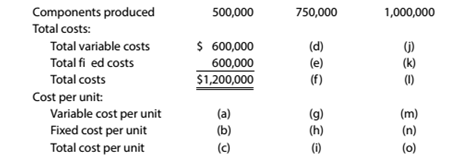Chapter 11, Problem 11.6E

Chapter
Section
Textbook Problem

Relevant range and fixed and variable costs Third World Gamer Inc. manufactures components for computer games within a relevant range of 500.000 to 1,000.000 disks per year. Within this range, the following partially completed manufacturing cost schedule has been prepared:Complete the cost schedule, identifying each cost by the appropriate letter (a) through (o).

To determine

Concept Introduction:

Variable, Fixed and Mixed Cost:

There are three types of costs according to the unit of production; Variable, Fixed and Mixed. Variable costs change proportionally with the number of units produced and variable cost per unit remains constant. Fixed Cost remains same in totality irrespective of the number of units produced. The mixed cost is the mix of variable and fixed cost, some of its part is fixed and some variable.

To Calculate:

The complete cost schedule

Explanation

The complete cost schedule is calculated as follows:

 Number of units Produced 500,000 750,000 1,000,000 Total Costs: Total Variable Costs \$ 600,000 \$ 900,000 (d) \$ 1,200,000 (j) (750000*1.2) (1000000*1.2) Total Fixed Costs \$ 600,000 \$ 600,000 (e) \$ 600,000 (k) Total Cos...

Still sussing out bartleby?

Check out a sample textbook solution.

See a sample solution

The Solution to Your Study Problems

Bartleby provides explanations to thousands of textbook problems written by our experts, many with advanced degrees!

Get Started